Decision Trees ::¶

Class is implemented only using numpy and visualization are shown using matplotlib. Code for class of decision tree is given in trees.py file

In :
import numpy as np
import matplotlib.pyplot as plt

Import classifier and regressor from given file trees.py¶

In :
from trees import ClassificationTree, RegressionTree

for dataset and spliting, we need sklearn (Optional, if you have your own data)¶

In :
from sklearn import datasets
from sklearn.model_selection import train_test_split

Classification Tree¶

Iris Dataset¶

Loading and spliting for training and testing

In :
X = data.data
y = data.target

feature_names = data.feature_names #Optional

Xt,Xs, yt, ys = train_test_split(X,y,test_size=0.3)

print(X.shape,y.shape, Xt.shape, yt.shape, Xs.shape, ys.shape)
(150, 4) (150,) (105, 4) (105,) (45, 4) (45,)

Fitting a model (displaying the tree building)¶

In :
clf = ClassificationTree()
clf.fit(Xt,yt,verbose=2,feature_names=feature_names)
Number of features:: 4
Number of samples :: 105
---------------------------------------
|Building the tree.....................
|-Feature::3_petal length (cm) Gain::0.9 thr::3.39
|
|->True branch (>>>)..
|--Feature::4_petal width (cm) Gain::0.75 thr::1.78
|
|-->True branch (>>>)..
|---Feature::3_petal length (cm) Gain::0.1 thr::4.90
|
|--->True branch (>>>)..
|---->{Leaf Node:: value: 2 }

|--->False branch (<<<)..
|---Feature::2_sepal width (cm) Gain::0.81 thr::3.20
|
|--->True branch (>>>)..
|---->{Leaf Node:: value: 1 }

|--->False branch (<<<)..
|--->{Leaf Node:: value: 2 }

|-->False branch (<<<)..
|--Feature::3_petal length (cm) Gain::0.22 thr::5.1
|
|-->True branch (>>>)..
|---Feature::1_sepal length (cm) Gain::0.92 thr::6.1
|
|--->True branch (>>>)..
|---->{Leaf Node:: value: 2 }

|--->False branch (<<<)..
|--->{Leaf Node:: value: 1 }

|-->False branch (<<<)..
|-->{Leaf Node:: value: 1 }

|->False branch (<<<)..
|->{Leaf Node:: value: 0 }

|
|.........................tree is buit!
---------------------------------------

Plotting the resulting tree¶

In :
plt.figure(figsize=(15,8))
clf.plotTree(show=True)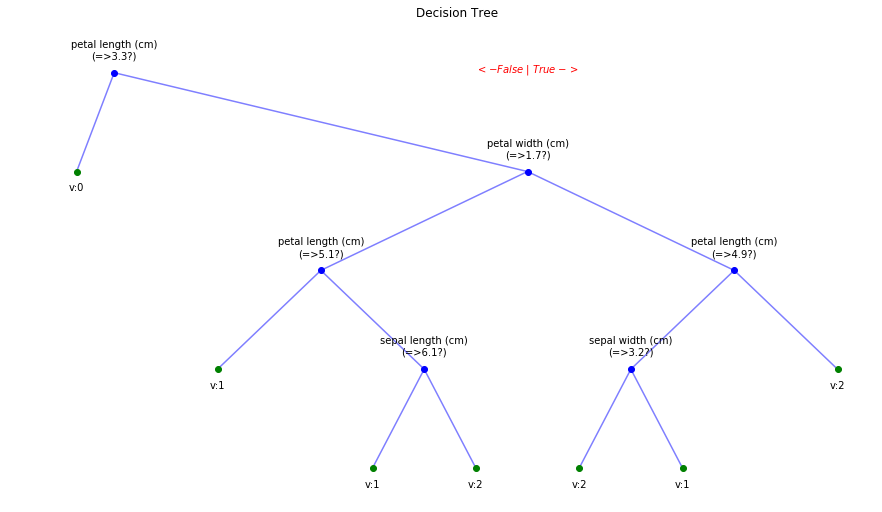Plotting Tree with color branches¶

In :
plt.figure(figsize=(15,8))
clf.plotTree(show=True,DiffBranchColor=True)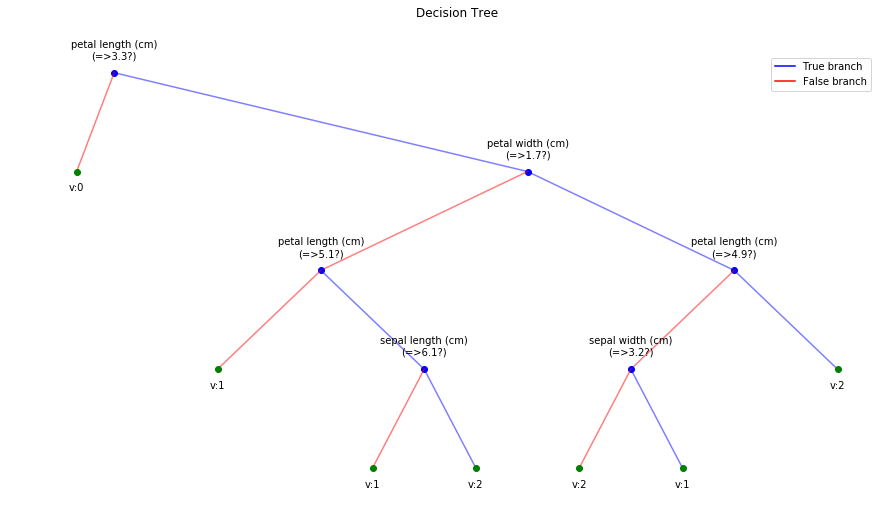Prediting and computing Accuracy¶

In :
ytp = clf.predict(Xt)
ysp = clf.predict(Xs)
print('Training Accuracy: ',np.mean(ytp==yt))
print('Testing  Accuracy: ',np.mean(ysp==ys))
Training Accuracy:  1.0
Testing  Accuracy:  0.9333333333333333

Iris data with smaller tree¶

In :
clf = ClassificationTree(max_depth=2)
clf.fit(Xt,yt,verbose=1,feature_names=feature_names)
#plt.figure(figsize=(15,8))
plt.figure(figsize=(7,6))
clf.plotTree(show=True)
ytp = clf.predict(Xt)
ysp = clf.predict(Xs)
print('Training Accuracy: ',np.mean(ytp==yt))
print('Testing  Accuracy: ',np.mean(ysp==ys))
Number of features:: 4
Number of samples :: 105
---------------------------------------
|Building the tree.....................
|subtrees::|100%|-------------------->||
|.........................tree is buit!
---------------------------------------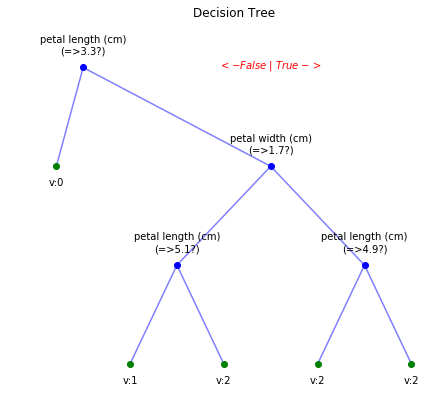Training Accuracy:  0.9809523809523809
Testing  Accuracy:  0.9333333333333333

Breast Cancer data¶

In :
X = data.data
y = data.target

feature_names = data.feature_names #Optional

Xt,Xs, yt, ys = train_test_split(X,y,test_size=0.3)

print(X.shape,y.shape, Xt.shape, yt.shape, Xs.shape, ys.shape)
(569, 30) (569,) (398, 30) (398,) (171, 30) (171,)

Fitting model with displaying the details of tree in process (verbose=2)¶

In :
clf = ClassificationTree()
clf.fit(Xt,yt,verbose=2,feature_names=feature_names)
Number of features:: 30
Number of samples :: 398
---------------------------------------
|Building the tree.....................
|-Feature::23_worst perimeter Gain::0.58 thr::115.905
|
|->True branch (>>>)..
|--Feature::27_worst concavity Gain::0.1 thr::0.219
|
|-->True branch (>>>)..
|--->{Leaf Node:: value: 0 }

|-->False branch (<<<)..
|--Feature::1_mean radius Gain::0.92 thr::16.5
|
|-->True branch (>>>)..
|--->{Leaf Node:: value: 1 }

|-->False branch (<<<)..
|-->{Leaf Node:: value: 0 }

|->False branch (<<<)..
|-Feature::28_worst concave points Gain::0.17 thr::0.11
|
|->True branch (>>>)..
|--Feature::22_worst texture Gain::0.22 thr::32.82
|
|-->True branch (>>>)..
|--->{Leaf Node:: value: 0 }

|-->False branch (<<<)..
|--Feature::21_worst radius Gain::0.27 thr::15.257
|
|-->True branch (>>>)..
|---Feature::22_worst texture Gain::0.44 thr::20.86
|
|--->True branch (>>>)..
|----Feature::8_mean concave points Gain::0.26 thr::0.05
|
|---->True branch (>>>)..
|-----Feature::14_area error Gain::0.37 thr::14.491.93
|
|----->True branch (>>>)..
|------>{Leaf Node:: value: 0 }

|----->False branch (<<<)..
|----->{Leaf Node:: value: 1 }

|---->False branch (<<<)..
|----Feature::16_compactness error Gain::0.95 thr::0.02
|
|---->True branch (>>>)..
|----->{Leaf Node:: value: 1 }

|---->False branch (<<<)..
|---->{Leaf Node:: value: 0 }

|--->False branch (<<<)..
|--->{Leaf Node:: value: 1 }

|-->False branch (<<<)..
|-->{Leaf Node:: value: 1 }

|->False branch (<<<)..
|-Feature::14_area error Gain::0.04 thr::45.3838:0.03
|
|->True branch (>>>)..
|--Feature::6_mean compactness Gain::0.97 thr::0.07
|
|-->True branch (>>>)..
|--->{Leaf Node:: value: 1 }

|-->False branch (<<<)..
|-->{Leaf Node:: value: 0 }

|->False branch (<<<)..
|-Feature::22_worst texture Gain::0.02 thr::33.37
|
|->True branch (>>>)..
|--Feature::22_worst texture Gain::0.39 thr::33.75
|
|-->True branch (>>>)..
|--->{Leaf Node:: value: 1 }

|-->False branch (<<<)..
|-->{Leaf Node:: value: 0 }

|->False branch (<<<)..
|->{Leaf Node:: value: 1 }

|
|.........................tree is buit!
---------------------------------------

Fitting model with displaying the progress only (verbose=1)¶

In :
clf = ClassificationTree()
clf.fit(Xt,yt,verbose=1,feature_names=feature_names)
Number of features:: 30
Number of samples :: 398
---------------------------------------
|Building the tree.....................
|subtrees::|100%|-------------------->|-
|.........................tree is buit!
---------------------------------------

Plotting Decison Tree¶

plt.figure(figsize=(15,8)) clf.plotTree(show=True)

Predicting and computing MSE¶

In :
ytp = clf.predict(Xt)
ysp = clf.predict(Xs)
print('Training Accuracy: ',np.mean(ytp==yt))
print('Testing  Accuracy: ',np.mean(ysp==ys))
Training Accuracy:  1.0
Testing  Accuracy:  0.9415204678362573

It's overfitting, try with smaller trees by decresing the max_depth of classifier

Regression Tree¶

Boston House price¶

In :
X = data.data
y = data.target

feature_names = data.feature_names #Optional

Xt,Xs, yt, ys = train_test_split(X,y,test_size=0.3)

print(X.shape,y.shape, Xt.shape, yt.shape, Xs.shape, ys.shape)
(506, 13) (506,) (354, 13) (354,) (152, 13) (152,)
In :
rgr = RegressionTree()
rgr.fit(Xt,yt,verbose=1,feature_names = feature_names)
Number of features:: 13
Number of samples :: 354
---------------------------------------
|Building the tree.....................
|subtrees::|100%|-------------------->|\
|.........................tree is buit!
---------------------------------------
In :
plt.figure(figsize=(15,15))
rgr.plotTree(show=True,scale=True, showtitle =False, showDirection=False,DiffBranchColor=True)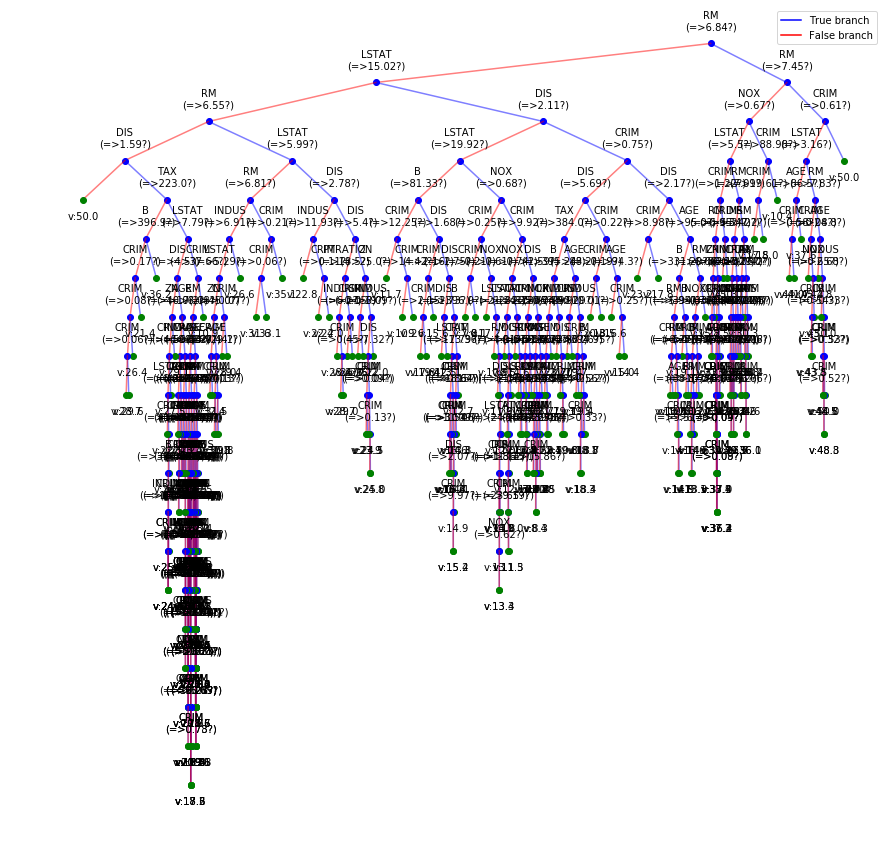In :
ytp = rgr.predict(Xt)
ysp = rgr.predict(Xs)
print('Training MSE: ',np.mean((ytp-yt)**2))
print('Testing  MSE: ',np.mean((ysp-ys)**2))
Training MSE:  0.0
Testing  MSE:  36.5921052631579

Boston Data with smaller tree¶

In :
rgr = RegressionTree(max_depth=3)
rgr.fit(Xt,yt,verbose=1,feature_names = feature_names)
Number of features:: 13
Number of samples :: 354
---------------------------------------
|Building the tree.....................
|subtrees::|100%|-------------------->|\
|.........................tree is buit!
---------------------------------------
In :
plt.figure(figsize=(15,8))
rgr.plotTree(show=True,scale=True, showtitle =True, showDirection=False,DiffBranchColor=True)

ytp = rgr.predict(Xt)
ysp = rgr.predict(Xs)
print('Training MSE: ',np.mean((ytp-yt)**2))
print('Testing  MSE: ',np.mean((ysp-ys)**2))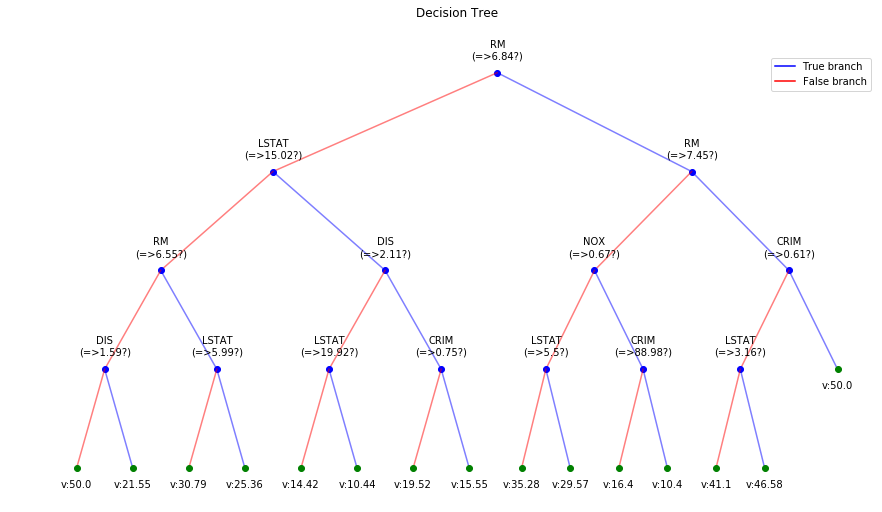Training MSE:  9.20127666641687
Testing  MSE:  36.44975827108703